Home Practice
For learners and parents For teachers and schools
Textbooks
Full catalogue
Pricing SupportLog in

We think you are located in United States. Is this correct?

# Chapter 17: Electric circuits

## 17.1 Potential difference and emf (ESAFA)

When a circuit is connected and complete, charge can move through the circuit. Charge will not move unless there is a reason, a force to drive it round the circuit. Think of it as though charge is at rest and something has to push it along. This means that work needs to be done to make charge move. A force acts on the charges, doing work, to make them move. The force is provided by the battery in the circuit.

A battery has the potential to drive charge round a closed circuit, the battery has potential energy that can be converted into electrical energy by doing work on the charge in the circuit to make it move.

Potential Difference

Potential difference is the work done per unit charge, $$\frac{W}{q}$$. The units of potential difference are the volt (V) which is defined as one joule per coulomb.

Quantity: Potential difference (V)        Unit name: volt       Unit symbol: V

temp text

### Voltmeter (ESAFB)

A voltmeter is an instrument for measuring the potential difference between two points in an electric circuit.

The symbol for a voltmeter is: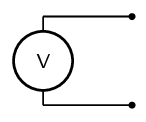A voltmeter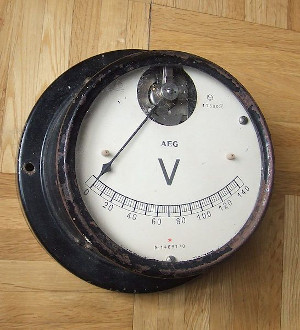temp text

### EMF (ESAFC)

When you measure the potential difference across (or between) the terminals of a battery that is not in a complete circuit you are measuring the emf of the battery. This is the maximum amount of work per coulomb of charge the battery can do to drive charge from one terminal, through the circuit, to the other terminal.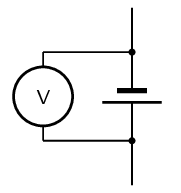The volt is named after the Italian physicist Alessandro Volta (1745–1827).

Electrical potential difference is also called voltage.

When you measure the potential difference across (or between) the terminals of a battery that is in a complete circuit you are measuring the terminal potential difference of the battery. Although this is measured in volts it is not identical to the emf. The difference will be the work done to drive charge through the battery.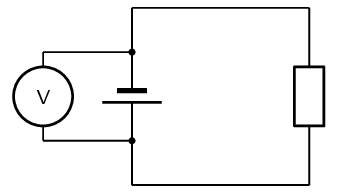Batteries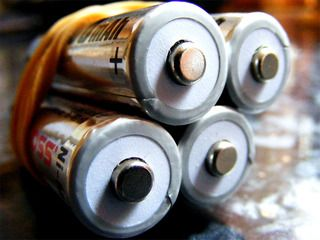Photography by scalespeeder on Flickr.com

One lead of the voltmeter is connected to one end of the battery and the other lead is connected to the opposite end. The voltmeter may also be used to measure the voltage across a resistor or any other component of a circuit but must be connected in parallel.